# Texture Synthesis Using Wavelets¶

Important: Please read the installation page for details about how to install the toolboxes. $\newcommand{\dotp}{\langle #1, #2 \rangle}$ $\newcommand{\enscond}{\lbrace #1, #2 \rbrace}$ $\newcommand{\pd}{ \frac{ \partial #1}{\partial #2} }$ $\newcommand{\umin}{\underset{#1}{\min}\;}$ $\newcommand{\umax}{\underset{#1}{\max}\;}$ $\newcommand{\umin}{\underset{#1}{\min}\;}$ $\newcommand{\uargmin}{\underset{#1}{argmin}\;}$ $\newcommand{\norm}{\|#1\|}$ $\newcommand{\abs}{\left|#1\right|}$ $\newcommand{\choice}{ \left\{ \begin{array}{l} #1 \end{array} \right. }$ $\newcommand{\pa}{\left(#1\right)}$ $\newcommand{\diag}{{diag}\left( #1 \right)}$ $\newcommand{\qandq}{\quad\text{and}\quad}$ $\newcommand{\qwhereq}{\quad\text{where}\quad}$ $\newcommand{\qifq}{ \quad \text{if} \quad }$ $\newcommand{\qarrq}{ \quad \Longrightarrow \quad }$ $\newcommand{\ZZ}{\mathbb{Z}}$ $\newcommand{\CC}{\mathbb{C}}$ $\newcommand{\RR}{\mathbb{R}}$ $\newcommand{\EE}{\mathbb{E}}$ $\newcommand{\Zz}{\mathcal{Z}}$ $\newcommand{\Ww}{\mathcal{W}}$ $\newcommand{\Vv}{\mathcal{V}}$ $\newcommand{\Nn}{\mathcal{N}}$ $\newcommand{\NN}{\mathcal{N}}$ $\newcommand{\Hh}{\mathcal{H}}$ $\newcommand{\Bb}{\mathcal{B}}$ $\newcommand{\Ee}{\mathcal{E}}$ $\newcommand{\Cc}{\mathcal{C}}$ $\newcommand{\Gg}{\mathcal{G}}$ $\newcommand{\Ss}{\mathcal{S}}$ $\newcommand{\Pp}{\mathcal{P}}$ $\newcommand{\Ff}{\mathcal{F}}$ $\newcommand{\Xx}{\mathcal{X}}$ $\newcommand{\Mm}{\mathcal{M}}$ $\newcommand{\Ii}{\mathcal{I}}$ $\newcommand{\Dd}{\mathcal{D}}$ $\newcommand{\Ll}{\mathcal{L}}$ $\newcommand{\Tt}{\mathcal{T}}$ $\newcommand{\si}{\sigma}$ $\newcommand{\al}{\alpha}$ $\newcommand{\la}{\lambda}$ $\newcommand{\ga}{\gamma}$ $\newcommand{\Ga}{\Gamma}$ $\newcommand{\La}{\Lambda}$ $\newcommand{\si}{\sigma}$ $\newcommand{\Si}{\Sigma}$ $\newcommand{\be}{\beta}$ $\newcommand{\de}{\delta}$ $\newcommand{\De}{\Delta}$ $\newcommand{\phi}{\varphi}$ $\newcommand{\th}{\theta}$ $\newcommand{\om}{\omega}$ $\newcommand{\Om}{\Omega}$

This numerical tour explores texture synthesis using wavelets.

Image synthesis is obtained by drawing an image at random that satisfies some modeling constraint, that are usually learned from a given exemplar texture.

In :
addpath('toolbox_signal')


## Multi-scale Texture Synthesis¶

The decay of wavelet coefficients caraterize pointwise singularities in images and texture. Histogram equalization enable the synthesis of texture with singularities. This corresponds to the texture synthesis algorithm of Heeger and Bergen.

In :
n = 512;
name = 'texture';
M = rescale( sum(M,3) );


For Scilab users: you should increase the size of the memory. Warning: execute this line only once.

In :
extend_stack_size(4);


First we compute the wavelet coefficients of the texture. We use a translation invariant transform.

In :
options.ti = 1;
Jmin = 4;
MW = perform_wavelet_transf(M(:,:,1), Jmin, +1, options);


We initialize the synthesis by a random noise with the same gray values.

In :
M1 = perform_hist_eq(randn(n,n), M);


Display.

In :
clf;
imageplot(M, 'Exemplar', 1,2,1);
imageplot(M1, 'Initial noise', 1,2,2);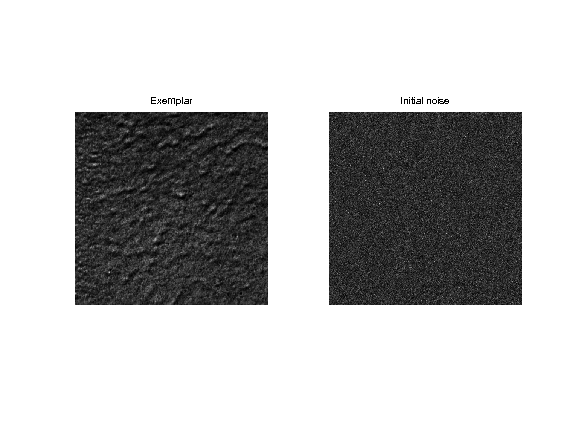We also compute the wavelet transform of the noise.

In :
MW1 = perform_wavelet_transf(M1, Jmin, +1, options);


A random texture is obtained by histogram equalization of each wavelet scale.

In :
for i=1:size(MW,3)
MW1(:,:,i) = perform_hist_eq(MW1(:,:,i), MW(:,:,i));
end


We retrieve the texture by inverse wavelet transform.

In :
M1 = perform_wavelet_transf(MW1, Jmin, -1, options);


Display.

In :
clf;
imageplot(M, 'Exemplar', 1,2,1);
imageplot(M1, 'Initial synthesis', 1,2,2);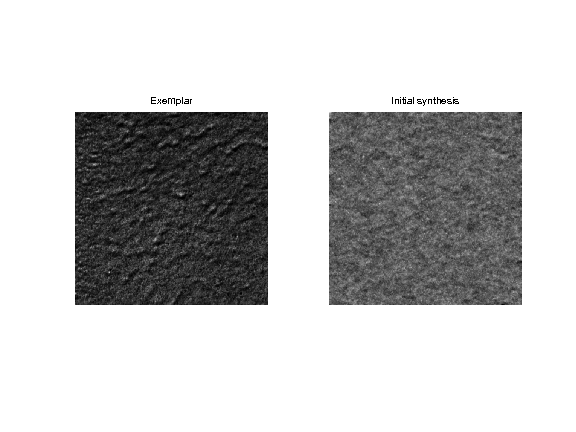Exercise 1

Iterate these two steps (spatial and wavelet histogram matching) until convergence to a stable step. pacial matching

In :
exo1()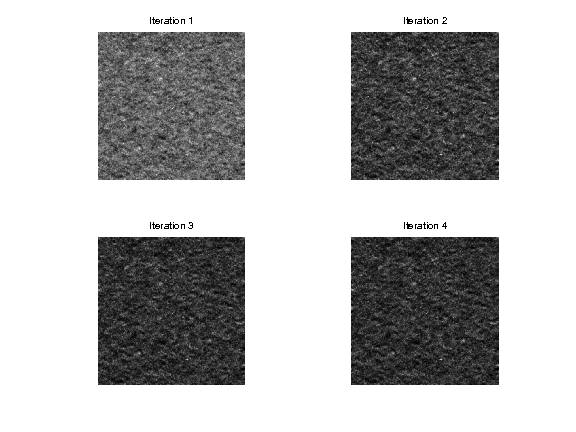In :
%% Insert your code here.


## Multi-scale Color Texture Synthesis¶

It is possible to perform color synthesis by synthesizing independantly each channel over a well chosen color space.

In :
n = 512;
M = rescale( load_image('texture', n) );


Exercise 2

Perform color texture synthesis with wavelets over the RGB space. isplay.

In :
exo2()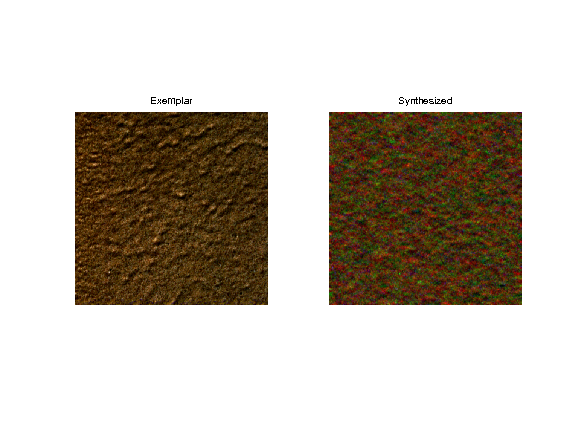In :
%% Insert your code here.


Exercise 3

Try with other color spaces, for instance PCA adapte space.

In :
exo3()

In :
%% Insert your code here.


## Multi-dimensional Color Equalization¶

To maintain color consistency, it is possible to use a color equalization.

Initial image.

In :
M1 = randn(n,n,3);


A simple (but not very acurate) method to perform in performing independant channel equalization over randomized color space. This needs to be repeated several time to converge to a real matching.

Compute a random 3x3 orthogonal matrix.

In :
[U,R] = qr(randn(3));


Perform the change of color space.

In :
d = reshape(M,[n^2 3])*U;
d1 = reshape(M1,[n^2 3])*U;


Perform the equalization

In :
for c=1:3
d1(:,c) = perform_hist_eq(d1(:,c),d(:,c));
end


Perform the inverse change of color space.

In :
M1 = reshape(d1*U',[n n 3]);


Compares the histogram of the R channel. You can see that the match is imperfect.

In :
m = M(:,:,1); m1 = M1(:,:,1);
clf;
subplot(2,1,1);
hist(m(:),50); title('Original');
subplot(2,1,2);
hist(clamp(m1(:)),50); title('Matched');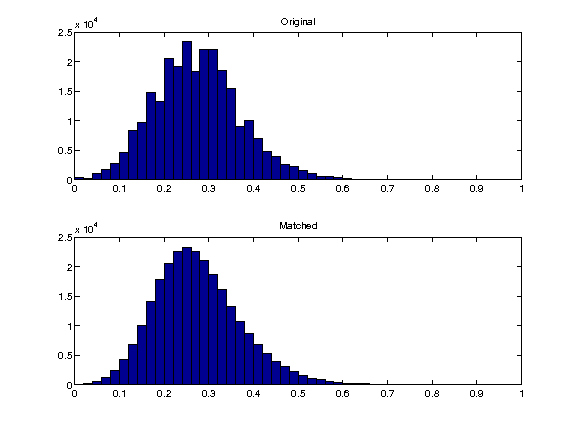In :
M1 = randn(n,n,3);
for i=1:3
M1(:,:,i) = perform_hist_eq(M1(:,:,i), M(:,:,i));
end


Exercise 4

Perform iteratively the randomized matching. Plot the decay of the mathing error.

In :
exo4()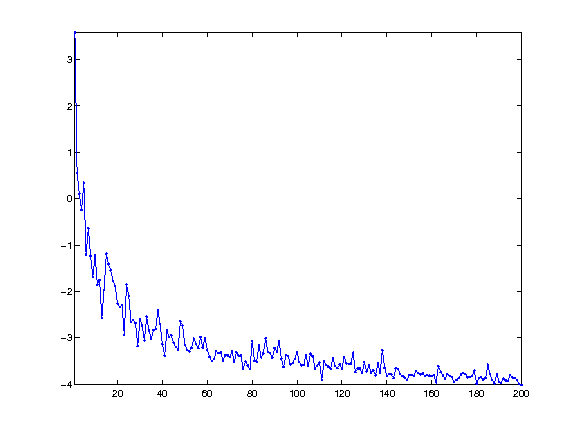In :
%% Insert your code here.


Display the histograms of the R channels. The match is not perfect, but it is better than with a single projection.

In :
m = M(:,:,1); m1 = M1(:,:,1);
clf;
subplot(2,1,1);
hist(m(:),50); title('Original');
subplot(2,1,2);
hist(clamp(m1(:)),50); title('Matched');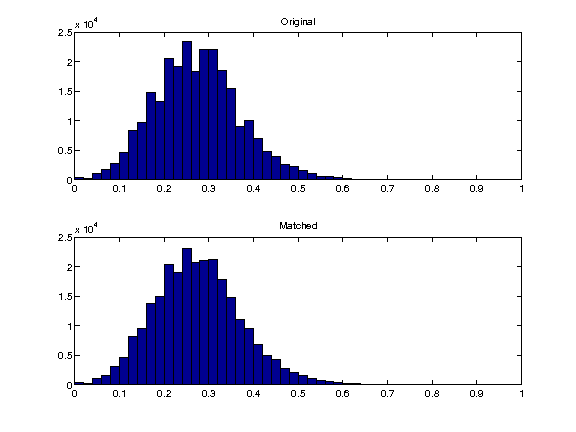Display the equalized color image;

In :
clf;
imageplot(M, 'Image', 1,2,1);
imageplot(M1, 'Equalized', 1,2,2);